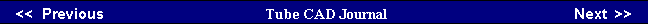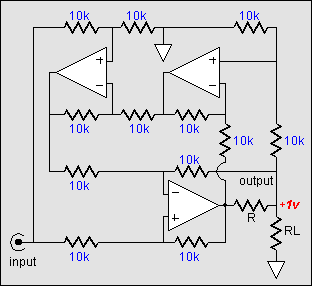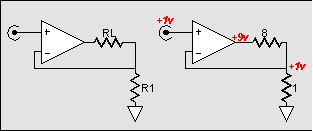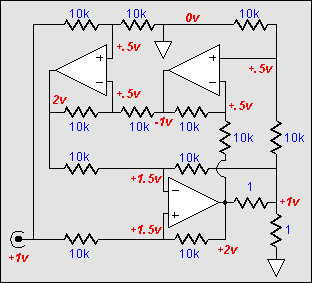How such as magic trick accomplished? Not easily, I fear. Feedback of both the current and the voltage into the load is taken and effectively multiplied against each other and whose product equals a constant defined by the input voltage across resistor R. The insertion of this small series resistor with the loudspeaker and the output allows a sampling of the current through the loudspeaker, while the voltage across the output can indirectly sampled through voltage dividers and Op-Amps. Ultimately, the two feedback voltages are summed and compared to the input voltage.
 This alternate model might be a natural for use with tubes as output devices, if the complexities of the function can be overcome, as tubes are not voltage shy. For example, if a tube OTL amplifier saw an increase impedance, it would have the easier task of giving up current for voltage. On the other hand, if the impedance declines, the tube OTL is in trouble, as it must give up voltage for current. But as most, but certainly not all, loudspeakers have an impedance that spikes up from a nominal base line, this last scenario might not be a problem the amplifier would have to face.     The second model is the voltage-to-current amplifier. Here an input voltage is translated into current. For example, a one volt input might equal 4 amperes of output current. What would be the output voltage? It depends entirely on the load. If the load were a dead short, the output voltage would be zero, although the full 4 amperes would be delivered into ground.Voltage-to-Power  amplifier
Wout = Vin² / R

Voltage-to-current amplifier
Iout = Vin / RThis amplifier is the inverse of the voltage-to-voltage amplifier to which we are so accustomed. Where the voltage amplifier is damaged by direct short to ground, as the amplifier strives to deliver infinite current into 0 ohms, the current amplifier is damaged by complete open circuits, as the amplifier strives to infinitely increase its output voltage to bring the current inline with its voltage-to-current ratio. Where the voltage amplifier has an output impedance approaching zero, the current amplifier has an output impedance approaching infinity. Obviously, pentodes hold a better hand in this regard compared to triodes, as the pentode enjoys a much higher plate impedance.